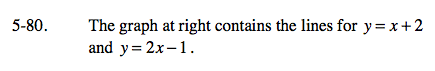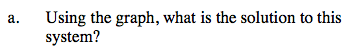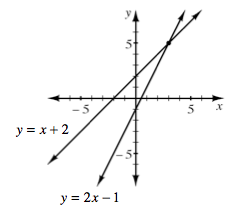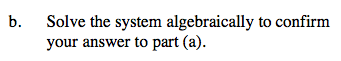### Home > CAAC > Chapter 5 > Lesson 5.2.2 > Problem5-80

5-80.What is the point at which the two lines intersect?

(3, 5)Use the Equal Values method by setting the equations equal to each other.

Did you get the same solutions for x and y as in part (a)?